# DC Models and Analysis

This section describes DC models you can use in EasyPower and describes the analysis results.

## DC Analysis Concepts

Please note the following about short circuit results:

• Short circuit currents are steady state approximations. Circuit inductance and capacitance are not considered and the buildup of currents is not modeled.
• Motors contribute to bus faults, but loads do not.
• Fault current from the rectifier at the rectifier terminal is specified in the rectifier dialog box.

### DC Motor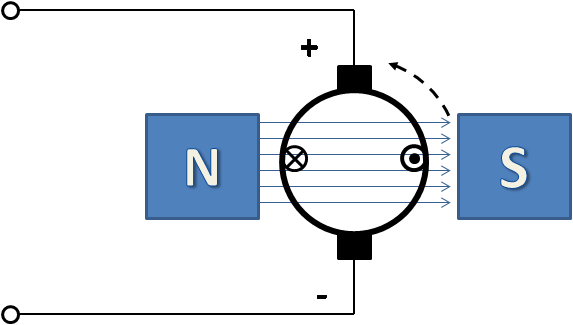Figure 1: DC Motor

The simplified electrical characteristic of the DC motor can be described by the following equation:

Vt = Ea + Ia * Ra

Where,

Vt = Terminal voltage

Ea = Induced armature voltage (back e.m.f.)

Ia = Armature current

Ra = Armature resistance

The factors affecting the induced armature voltage are:

• Magnetic flux
• Number of armature conductors
• Speed

DC motor starting: While starting DC motors, speed is initially zero and so Ea is zero. As a result, the starting current is high. The starting current can be calculated from the equation: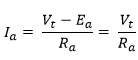DC motor short circuit contribution: The speed is assumed at rated speed, so Ea is close to the system voltage. The terminal voltage Vt collapses to near zero for fault near the terminal. The negative sign represents current flow out of the motor.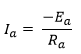The impedance Ra is used as the short circuit model in the calculations.

#### Shunt Motor Starting Example

Motor Data: HP = 100; V = 250; Efficiency = 95%; Ra = 0.05 ohms

FLA = Input kW / V = HP * 0.746 / (Efficiency * kV)

= 310 Amps

Starting Current = V / Ra

= 250 / 0.05 = 5000 Amps

Starting Resistor to limit current to 4 x FLA:

Rtotal = Ra + Rstart

Rtotal = V / (4 x FLA) = 250 / (4 x 310) = 0.20 ohms

Rstart = 0.20 – 0.05 = 0.15 ohms

Short Circuit Contribution to terminal fault:

~ 250 / 0.05 ~ 5000 Amps

#### Estimate of Armature Resistance

It is best to use the armature resistance from the manufacturer if it is available. The armature resistance needs to include the commutator resistance. In the absence of exact information, we can make some assumptions. The graph below shows the calculated percent impedance of a few DC motors obtained from a facility.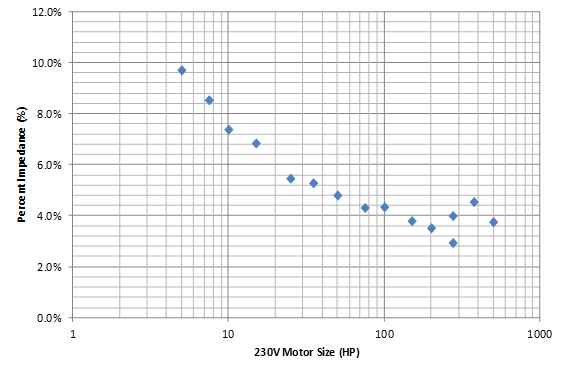Figure 2: Calculated Percent Impedances for Sample 230V DC Motors

For a motor rated at 50HP, 230V, 0.9 efficiency, we will estimate the armature resistance. For this example, assume 8% impedance. This is how much voltage drop there is across the armature and commutator at full load.

Vdrop = 8% of 230V = 18.4V at FLA.

FLA = 0.746 * HP / (Efficiency * V) = 180A

Ra = Vdrop / FLA = 18.4 / 180 = 0.1 Ohms

### Battery

A battery is modeled as a voltage source and a series resistance (internal resistance). This is true for both short circuit and power flow analysis.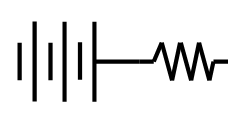Figure 3: Battery Electrical Model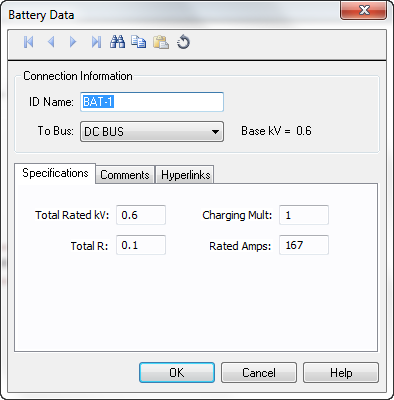Figure 4: Battery Dialog Box

Option Description
Total Rated kV This is the total voltage rating of the battery string. If you have multiple batteries connected in series, add up the voltage of individual batteries. For parallel connection for similar batteries, leave the voltage as is.
Charging Multiplier This is multiplier for the battery voltage reflecting the charge condition. For example, a fully charged battery could have 1.05 and a slightly drained battery could have 0.95 as the charging multiplier.
Total R

This is the internal resistance of the battery string in ohms. You can get the internal resistance from the manufacturer. Here is some typical data for batteries:

 Battery Type Percent Impedance Flooded 10-15% Gel 12-16% AGM 2%
Rated Amps The rated (nominal) amperes of the battery or battery string. The nominal amp rating is the ampere-hour divided by the discharge time for such batteries. For example, if you have a 200Amp-hour battery rated at a discharge time of 8 hours, the rated amps is 200 / 8 = 25A.

#### Example Battery Resistance Calculation

A flooded lead acid battery has a rating of 250V and 200Amp-hour with a discharge time of 8 hours. Estimate the resistance of the battery.

Rated Amps = 200 / 8 = 25A.

We will assume 10% impedance for this case.

Total R = V * (% Impedance) / (Rated Amps) = 250 * 0.1 / 25 = 1 Ohm.

#### Battery Bank

Multiple identical cells or batteries can be connected in series, in parallel, or in a combination of both.

• Parallel Connection: Current capacity is multiplied by the number of battery strings in parallel. Battery resistance is divided by the number of parallel connections. The voltage remains the same.
• Series Connection: For a string of batteries in series, the current capacity remains the same. Total voltage is the individual voltage times the number of batteries in series. The battery resistance is the sum of the individual resistances.

If you have the impedance in percent, there is no need to add or divide the percent value. You can calculate the resistance based on the final voltage and current capacity.

### Photovoltaics

The graph below shows an approximation of the V-I characteristics of photovoltaics. This is nonlinear and is used when solving power flow. As the load demand increases beyond the capacity of the photovoltaic, the voltage drops rapidly.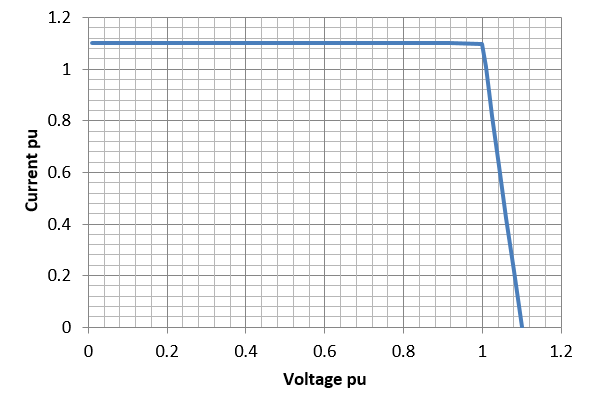Figure 5: V-I Characteristics of Photovoltaics

The characteristics are derived from the user defined inputs in the dialog box. The important data include:

• Open circuit voltage: This defines where the curve meets the voltage axis to the right.
• Short Circuit Current: This defines where the curve meets the current axis on the left.
• Voltage at which peak power is delivered: This defines the "knee" of the curve.

For more information about photovoltaics, see Example 3: Photovoltaic System.

### DC Arc Flash Hazard Analysis

NFPA 70E, Edition 2015, Annex D.5 describes the method for calculating arc flash hazard energy for low voltage DC equipment. This method is based on the maximum power transfer theorem similar to Ralph Lee's method for AC.

Iarc = 0.5 * Ibf

Ei = 0.01 * Iarc * V * t * Factor / D2

Where,

Iarc = estimated DC arc current in amps

Ei = estimated incident energy in cal/cm2 at the working distance D

V = DC voltage in volts

t = arc duration in seconds

D = working distance in cm

Factor = 1.0 for open air and 3.0 for enclosures (box)

 DC in EasyPower Example 2: DC Link Inverter with a Battery Bank (UPS) Tips on Creating DC Systems Example 3: Photovoltaic System Example 1: Sample DC System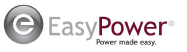EasyPower, LLC

7730 SW Mohawk St.

Tualatin, OR 97062

(503)655-5059

EasyPower Version 10.2